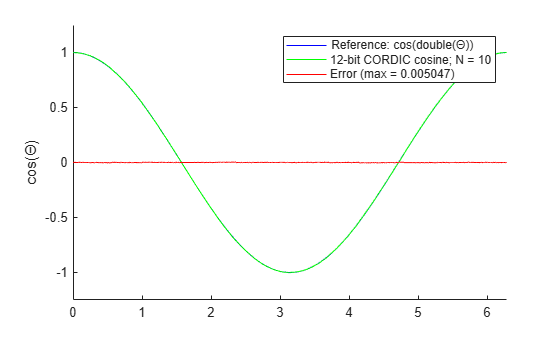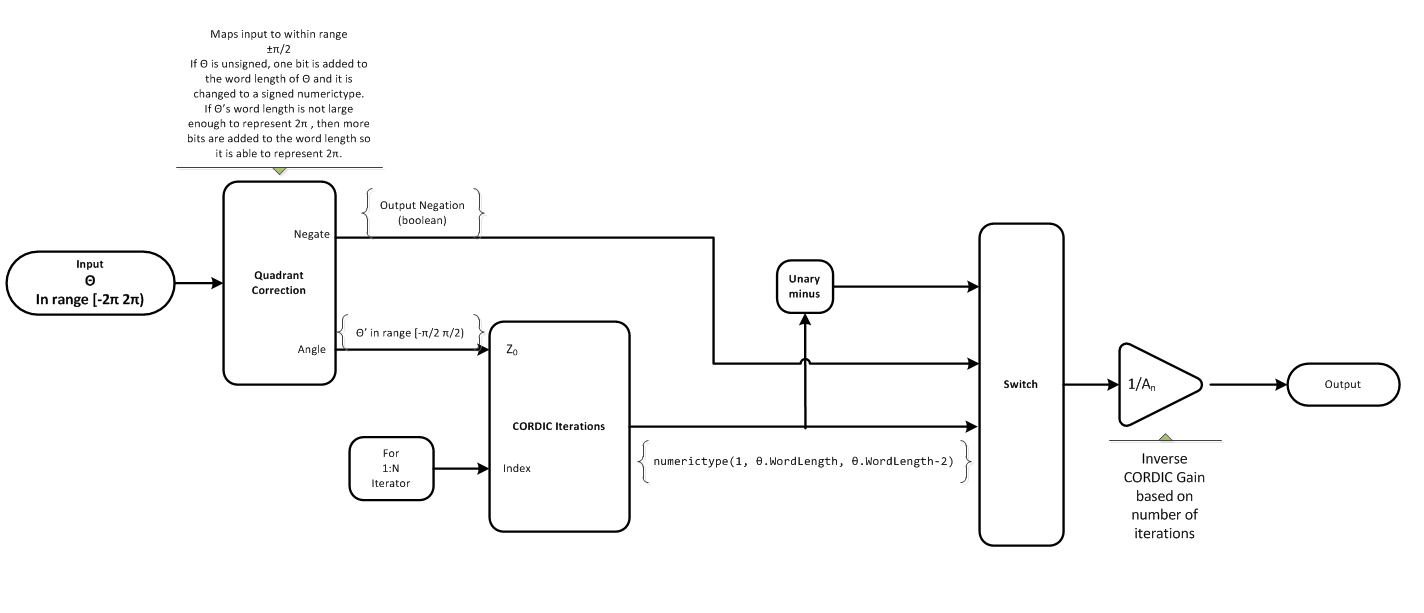# cordiccos

CORDIC-based approximation of cosine

## Syntax

```y = cordiccos(theta, niters) ```

## Description

`y = cordiccos(theta, niters)` computes the cosine of `theta` using a CORDIC algorithm approximation.

## Input Arguments

 `theta` `theta` can be a signed or unsigned scalar, vector, matrix, or `N`-dimensional array containing the angle values in radians. All values of `theta` must be real and in the range [–2π 2π). `niters` `niters` is the number of iterations the CORDIC algorithm performs. This is an optional argument. When specified, `niters` must be a positive, integer-valued scalar. If you do not specify `niters` or if you specify a value that is too large, the algorithm uses a maximum value. For fixed-point operation, the maximum number of iterations is one less than the word length of `theta`. For floating-point operation, the maximum value is 52 for double or 23 for single. Increasing the number of iterations can produce more accurate results, but it also increases the expense of the computation and adds latency.

## Output Arguments

 `y` `y` is the CORDIC-based approximation of the cosine of `theta`. When the input to the function is floating point, the output data type is the same as the input data type. When the input is fixed point, the output has the same word length as the input, and a fraction length equal to the `WordLength` – `2`.

## Examples

collapse all

Compare the results produced by various iterations of the `cordiccos` algorithm to the results of the double-precision `cos` function.

```% Create 1024 points between [0,2*pi) stepSize = pi/512; thRadDbl = 0:stepSize:(2*pi - stepSize); thRadFxp = sfi(thRadDbl,12); % signed, 12-bit fixed-point cosThRef = cos(double(thRadFxp)); % reference results % Use 12-bit quantized inputs and vary the number % of iterations from 2 to 10. % Compare the fixed-point CORDIC results to the % double-precision trig function results. for niters = 2:2:10 cdcCosTh = cordiccos(thRadFxp,niters); errCdcRef = cosThRef - double(cdcCosTh); end figure hold on axis([0 2*pi -1.25 1.25]); plot(thRadFxp,cosThRef,'b'); plot(thRadFxp,cdcCosTh,'g'); plot(thRadFxp,errCdcRef,'r'); ylabel('cos(\Theta)'); gca.XTick = 0:pi/2:2*pi; gca.XTickLabel = {'0','pi/2','pi','3*pi/2','2*pi'}; gca.YTick = -1:0.5:1; gca.YTickLabel = {'-1.0','-0.5','0','0.5','1.0'}; ref_str = 'Reference: cos(double(\Theta))'; cdc_str = sprintf('12-bit CORDIC cosine; N = %d',niters); err_str = sprintf('Error (max = %f)', max(abs(errCdcRef))); legend(ref_str,cdc_str,err_str);```After 10 iterations, the CORDIC algorithm has approximated the cosine of `theta` to within 0.005187 of the double-precision cosine result.

collapse all

### CORDIC

CORDIC is an acronym for COordinate Rotation DIgital Computer. The Givens rotation-based CORDIC algorithm is one of the most hardware-efficient algorithms available because it requires only iterative shift-add operations (see References). The CORDIC algorithm eliminates the need for explicit multipliers. Using CORDIC, you can calculate various functions, such as sine, cosine, arc sine, arc cosine, arc tangent, and vector magnitude. You can also use this algorithm for divide, square root, hyperbolic, and logarithmic functions.

Increasing the number of CORDIC iterations can produce more accurate results, but doing so also increases the expense of the computation and adds latency.

## Algorithms

collapse all

### Signal Flow DiagramsCORDIC Rotation KernelX represents the sine, Y represents the cosine, and Z represents theta. The accuracy of the CORDIC rotation kernel depends on the choice of initial values for X, Y, and Z. This algorithm uses the following initial values:

### fimath Propagation Rules

CORDIC functions discard any local `fimath` attached to the input.

The CORDIC functions use their own internal `fimath` when performing calculations:

• `OverflowAction``Wrap`

• `RoundingMethod``Floor`

The output has no attached `fimath`.

## References

 Volder, JE. “The CORDIC Trigonometric Computing Technique.” IRE Transactions on Electronic Computers. Vol. EC-8, September 1959, pp. 330–334.

 Andraka, R. “A survey of CORDIC algorithm for FPGA based computers.” Proceedings of the 1998 ACM/SIGDA sixth international symposium on Field programmable gate arrays. Feb. 22–24, 1998, pp. 191–200.

 Walther, J.S. “A Unified Algorithm for Elementary Functions.” Hewlett-Packard Company, Palo Alto. Spring Joint Computer Conference, 1971, pp. 379–386. (from the collection of the Computer History Museum). www.computer.org/csdl/proceedings/afips/1971/5077/00/50770379.pdf

 Schelin, Charles W. “Calculator Function Approximation.” The American Mathematical Monthly. Vol. 90, No. 5, May 1983, pp. 317–325.

## Extended Capabilities

### Topics

Introduced in R2010a

## SupportGet trial now